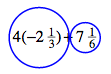Home > CC2MN > Chapter cc23 > Lesson cc23.2.3 > Problem3-61

3-61.

Simplify each of the following expressions without using a calculator. Draw diagrams or use words to show your thinking.  Homework Help ✎1. $4 ( - 2 \frac { 1 } { 3 } ) + 7 \frac { 1 } { 6 }$

Remember that terms are separated by addition symbols ($+$).

Can you draw or explain how you separate these terms? You should simplify these terms before adding them together.1. $- 8 + - 2 ( 3 )$

Adding negative numbers can be tricky. Could it be helpful to draw a diagram like the tightropes used in previous lessons?

The expression simplifies to $−14$.

1. $4 + - 5 ( - 3 ) + ( - 7 )$

Reminder: Multiplying two negative numbers results in a positive number. Can you find the product and explain why this is so?

The expression simplifies to $12$. Don't forget to show your thinking!

1. $( - 4.25 ) ( 2 ) + ( - 4.25 ) ( - 2 )$